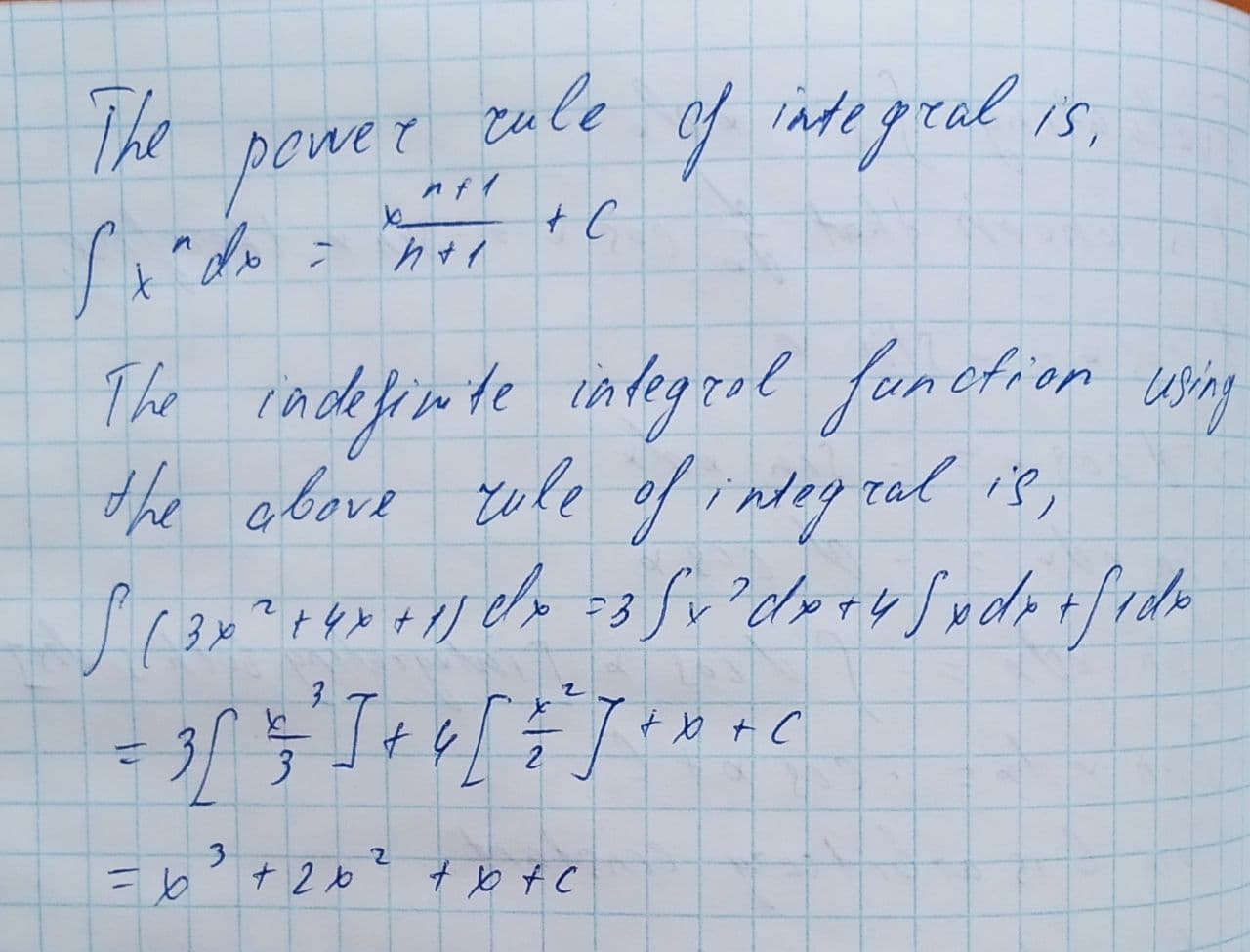Question# Find the general indefinite integral: \int (3x^{2}+4x+1)dx

Applications of integrals
ANSWEREDFind the general indefinite integral.
$$\displaystyle\int{\left({3}{x}^{{{2}}}+{4}{x}+{1}\right)}{\left.{d}{x}\right.}$$